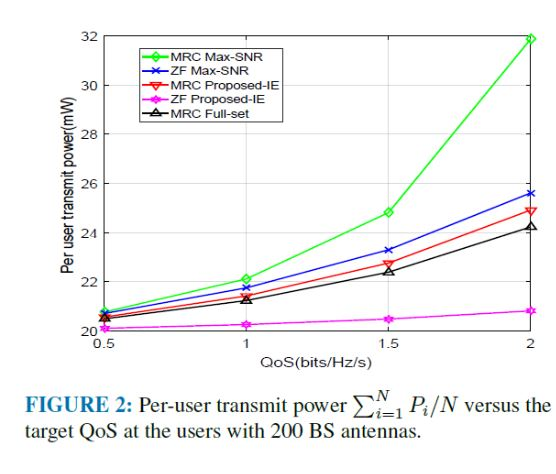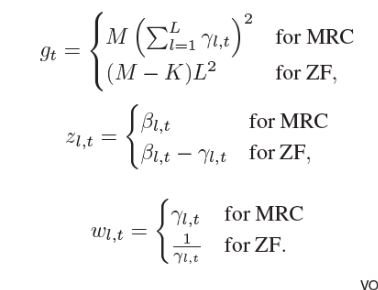# Errors in simulate the GP

Hi

I wanna simulate this GP in MATLAB
I try to model this, but there is some errors that I can’t fix it.

``````clear all;
clc;
N_antenna=200; %number of antennas
N_usersr=20; %number of users
N_BS=4; %number of B.Ss
loop=3;
noise_dB=-96; %in dBm
noise=10^(noise_dB/10);
P_max_dB=23; %in dBm
Pmax=10^(P_max_dB/10);
tau_p=N_usersr;
tau_c=200;  %coherent interval
QoS=landa=0.5:0.5:2; %bit/Hz/s
%nbrOfRealizations=10;
Pp=200; %pilot power in mW
%QoS=2.^(QoS*tau_c/(tau_c-tau_p))-1;
%%
Comp_MRT_fixNa=zeros(length(QoS),N_usersr);
Comp_ZF_fixNa=zeros(length(QoS),N_usersr);
for iter=1:loop
%generate BS location
for i=1:4
BS_locations(i,:)=[cos(i*pi/2) sin(i*pi/2)]/2;
end

%generate UE location
rng('shuffle'); %Initiate the random number generators with a random seed

minimal_dist=0.035;

%for iter =1: nbrOfRealizations
%    iter
UserLocationLargeScale = zeros(N_usersr,1);
% Step 1: Generate user locations
% Uniformly distributed users in the network
distance_normalized = 2*minimal_dist+sqrt(rand(N_usersr,1));
angles = rand(N_usersr,1)*2*pi;
%%
% Generate MS locations
% Tolerance to stopping optimizat
%    MSlocationSave(:,:,iter)=MSlocations;
MSlocationSave=MS_locations;

for k=1:N_usersr
dista(k,:) = sqrt(sum(abs(BS_locations -repmat(MS_locations(k,:),[N_BS 1])).^2,2));

% Go through all transmitters
for j=1 :N_BS
end
gamma=tau_p*Pp.*(beta).^2./(tau_p*Pp.*beta+noise);
end
[value ind]=min(dista');

for k=1:length(QoS)
%% code for optimizing max snr MRT
cvx_begin gp
cvx_quiet true
variables PmaxsnrMRT(N_usersr) landa %nonnegative
maximize landa
subject to
for i=1:N_usersr
10^22*(PmaxsnrMRT(i).*gamma(i,ind(i))*200) >= 10^22*(landa*(noise+sum(PmaxsnrMRT'*(beta))));
PmaxsnrMRT(i)<= Pmax;
PmaxsnrMRT(i) >=0;

end
cvx_end
PfixQoS_maxSnr(iter,k)=sum(PmaxsnrMRT);
end
end
save('PfixQoS_maxSnr')``````
1 Like

The code before CVX is invoked is not even valid MATLAB code.
`QoS=landa=0.5:0.5:2; %bit/Hz/s`

Please make sure the code runs. And show us what the problem is. Does CVX accept the code? Doe the solver solve it? Does it fail only in later CVX invocations? Remove the `quiet`option so that you can see the solver and CVX output.

Why do you multiply both sides of an inequality by 10^22? I doubt that’s a good thing to do. Huge or small log values of orders of magnitude 9from magnitude 1) will likely cause solver difficulties.

QoS=landa=0.5:0.5:2; %bit/Hz/s
landa is the CVX variable and above code is incorrect.
The correct state of the code is:
QoS=0.5:0.5:2; %bit/Hz/s.

The purpose of this code is to extract the green diagram in the figure belowThe code runs correctly but all of the CVX output answers are the same and don’t vary with the QoS changes.
I use 10^22 to scale up both sides of inequality and I Definitely don’t know that is correct or not.!!!

I think there is major problems with writing code loops and also CVX variables, which I can’t fix it
I’ll be grateful if you guide me.

Also in the first pic SINR coefficients are as:That all of them are scaller and vary for each user (t)

You need sensible input values and good numerical scaling (choice of units), otherwise your problem may not be solved reliably. That could explain lack of sensitivity of results to some specific input, if something important in the model is being drowned out.

I have no idea what your model does, or what are sensible results. Nevertheless, there is some variation of result across the iterations. Here is output for one set of iterations (corresponding to the random numbers drawn that time)

``````       iter  k   landa
1    1   7.5693
1    2   7.5693
1    3   7.5693
1    4   7.5693
2    1   8.4597
2    2   8.4597
2    3   8.4597
2    4   8.4597
3    1   7.9611
3    2   7.9611
3    3   7.9611
3    4   7.9611
``````

BTW, I haven’t checked whether you’ve implemented the model correctly (leaving aside scaling).

In the first pic in this topic has been said that QoS of t-th user is equivalent SINR.
and relation between R_t and SINR_t is as:
R_t >= constant * log_2(1 + SINR_t)

According to 39 and 40 equations in first pic, and according to this fact that QoS vary 0.5 to 2 in figure, ** I have to include QoS variation in CVX code but I don’t know where to put it!? **
If possible, check out the 39 and 40 equations with CVX code Considering QoS changes.

It looks like the variation in the 12 runs which you are performing is because of choosing different random inputs. The for loop, `for k=1:length(QoS)` is running the exact same CVX problem `length(QoS)` times. There appears to be nowhere in your program that `QoS` is “used” It is set in the statement `QoS=landa=0.5:0.5:2;` and then is never used, other than its length determining how many times the for loop, `for k=1:length(QoS)` is executed.

landa is a CVX variable and it was written wrong and isn’t same with QoS and `QoS=0.5:0.5:2;`
don’t you have any idea about putting QoS in CVX program.!?
QoS is the Horizontal axis in figure.
I really don’t know how to solve this problem.

I have no knowledge of the subject matter, nor the meaning of the various inputs… If QoS is supposed to affect the optimal solution, then it should somehow, directly, or indirectly, enter into the input data to the CVX optimization problem. That is not a CVX issue, it is a model formulation issue which is your responsibility.

Your program defines a QoS array, and then never uses it.